# Plots and Trend Lines 4 5 Scatter Plots

• Slides: 15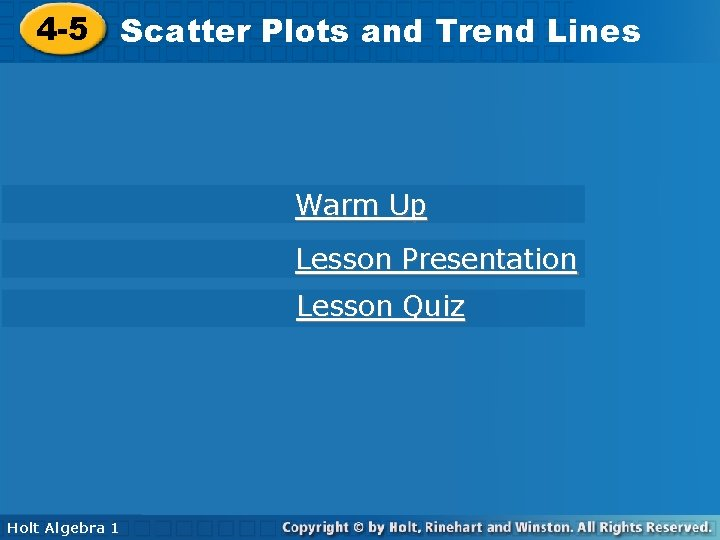Plots and Trend Lines 4 -5 Scatter Plots and Trend Lines Warm Up Lesson Presentation Lesson Quiz Holt Algebra 1 1 Holt Algebra4 -5 Scatter Plots and Trend Lines Warm Up Graph each point. 1. A(3, 2) 3. C(– 2, – 1) 5. E(1, 0) Holt Algebra 1 2. B(– 3, 3) 4. D(0, – 3) 6. F(3, – 2)4 -5 Scatter Plots and Trend Lines Objectives Create and interpret scatter plots. Use trend lines to make predictions. Holt Algebra 14 -5 Scatter Plots and Trend Lines Vocabulary scatter plot correlation positive correlation negative correlation no correlation trend line Holt Algebra 1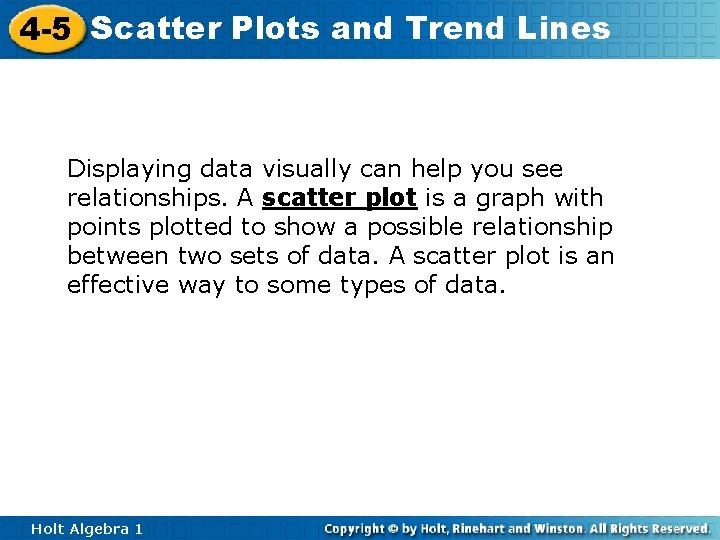4 -5 Scatter Plots and Trend Lines Displaying data visually can help you see relationships. A scatter plot is a graph with points plotted to show a possible relationship between two sets of data. A scatter plot is an effective way to some types of data. Holt Algebra 1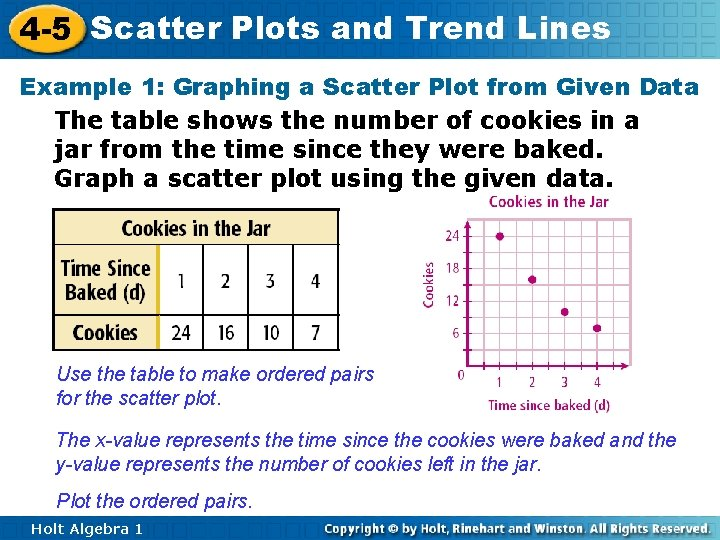4 -5 Scatter Plots and Trend Lines Example 1: Graphing a Scatter Plot from Given Data The table shows the number of cookies in a jar from the time since they were baked. Graph a scatter plot using the given data. Use the table to make ordered pairs for the scatter plot. The x-value represents the time since the cookies were baked and the y-value represents the number of cookies left in the jar. Plot the ordered pairs. Holt Algebra 1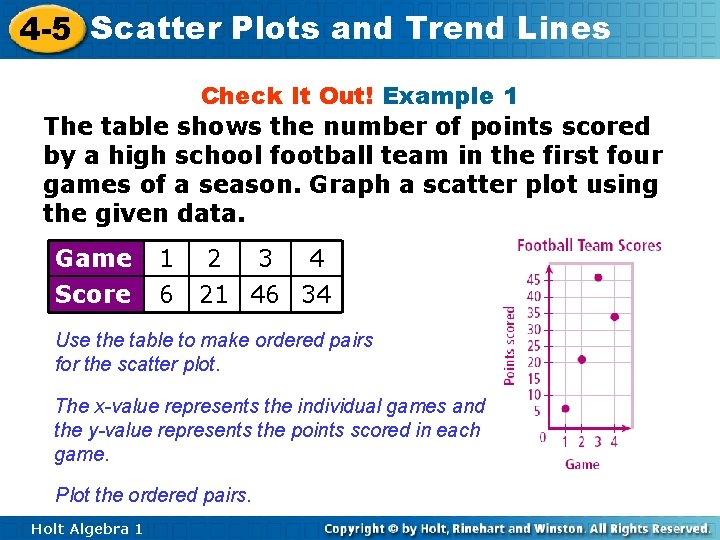4 -5 Scatter Plots and Trend Lines Check It Out! Example 1 The table shows the number of points scored by a high school football team in the first four games of a season. Graph a scatter plot using the given data. Game Score 1 6 2 3 4 21 46 34 Use the table to make ordered pairs for the scatter plot. The x-value represents the individual games and the y-value represents the points scored in each game. Plot the ordered pairs. Holt Algebra 14 -5 Scatter Plots and Trend Lines A correlation describes a relationship between two data sets. A graph may show the correlation between data. The correlation can help you analyze trends and make predictions. There are three types of correlations between data. Holt Algebra 14 -5 Scatter Plots and Trend Lines Holt Algebra 14 -5 Scatter Plots and Trend Lines Example 2: Describing Correlations from Scatter Plots Describe the correlation illustrated by the scatter plot. As the average daily temperature increased, the number of visitor increased. There is a positive correlation between the two data sets. Holt Algebra 1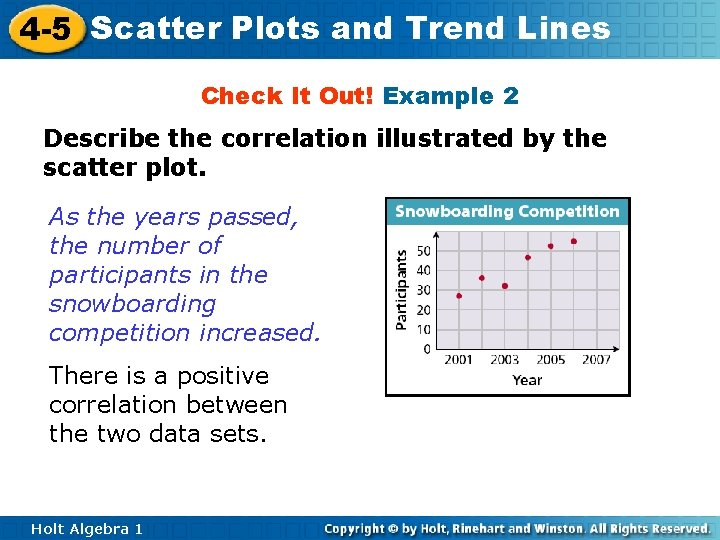4 -5 Scatter Plots and Trend Lines Check It Out! Example 2 Describe the correlation illustrated by the scatter plot. As the years passed, the number of participants in the snowboarding competition increased. There is a positive correlation between the two data sets. Holt Algebra 1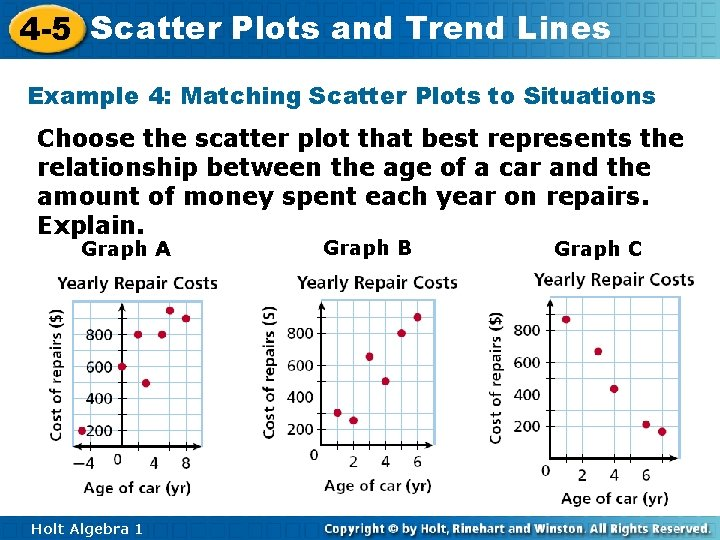4 -5 Scatter Plots and Trend Lines Example 4: Matching Scatter Plots to Situations Choose the scatter plot that best represents the relationship between the age of a car and the amount of money spent each year on repairs. Explain. Graph A Holt Algebra 1 Graph B Graph C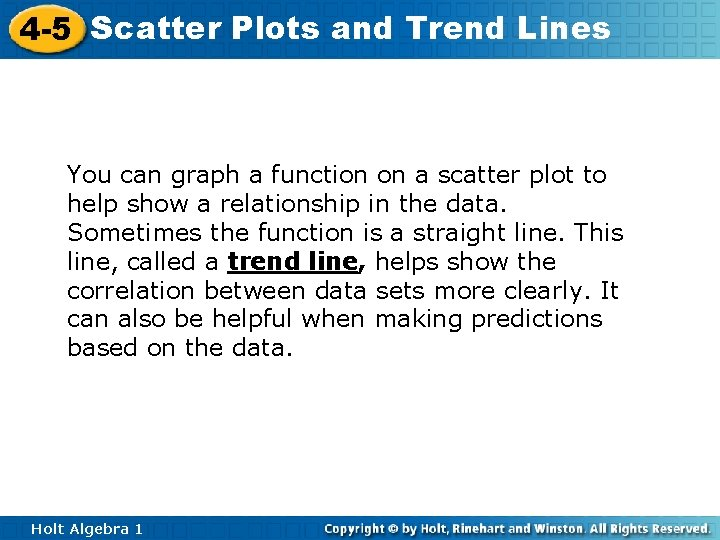4 -5 Scatter Plots and Trend Lines You can graph a function on a scatter plot to help show a relationship in the data. Sometimes the function is a straight line. This line, called a trend line, helps show the correlation between data sets more clearly. It can also be helpful when making predictions based on the data. Holt Algebra 1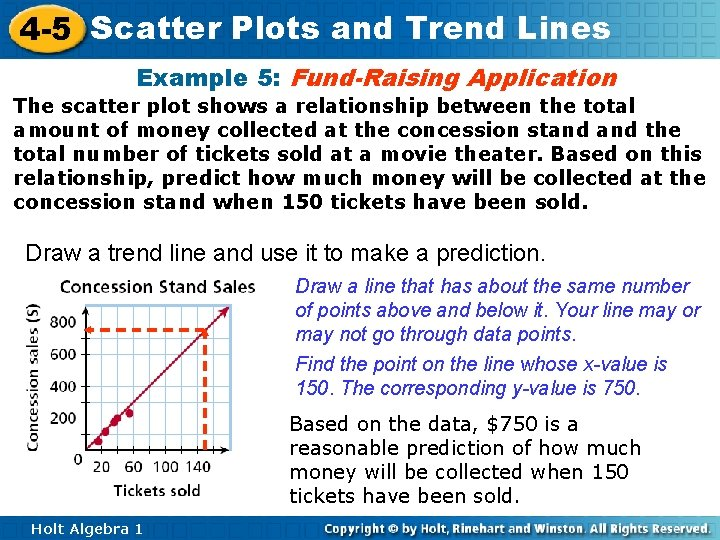4 -5 Scatter Plots and Trend Lines Example 5: Fund-Raising Application The scatter plot shows a relationship between the total amount of money collected at the concession stand the total number of tickets sold at a movie theater. Based on this relationship, predict how much money will be collected at the concession stand when 150 tickets have been sold. Draw a trend line and use it to make a prediction. Draw a line that has about the same number of points above and below it. Your line may or may not go through data points. Find the point on the line whose x-value is 150. The corresponding y-value is 750. Based on the data, \$750 is a reasonable prediction of how much money will be collected when 150 tickets have been sold. Holt Algebra 14 -5 Scatter Plots and Trend Lines Check It Out! Example 5 Based on the trend line, predict how many wrapping paper rolls need to be sold to raise \$500. Find the point on the line whose y-value is 500. The corresponding x-value is about 75. Based on the data, about 75 wrapping paper rolls is a reasonable prediction of how many rolls need to be sold to raise \$500. Holt Algebra 1